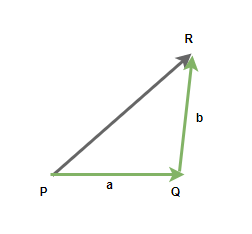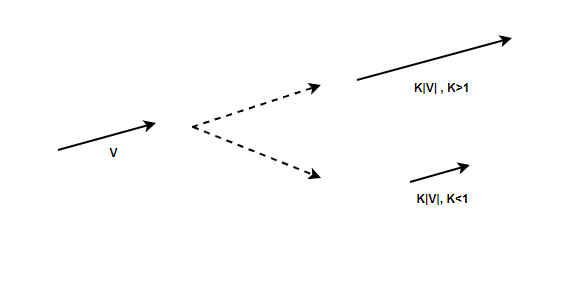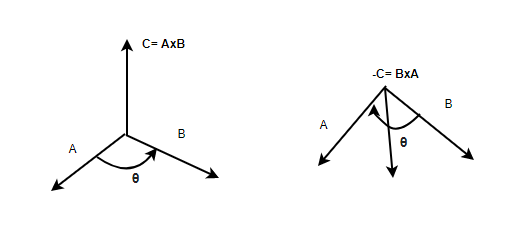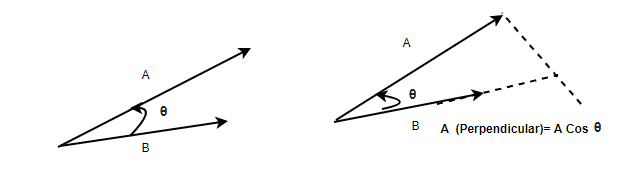GFG App
Open AppBrowser
Continue

In physics, there are mainly two types of quantities – Vectors and Scalars. Vectors are quantities that have both direction and magnitude associated with them while scalars are the quantities that have the only magnitude associated with them. Scalar quantities can be dealt with using simple algebraic rules but this is not the case with vector quantities, they cannot be dealt with in the same way. Thus, It is essential to know what kind of and how many different operations can be performed on these quantities. Let’s look at some of these operations in detail.

### Mathematical Operations on Vectors

Since vectors contain directions, these quantities should be dealt with in ways that take into account their directions. The basic rules of algebra do not apply in general for vectors, for example – a simple addition of the magnitudes of the two vectors will give the wrong answer in most cases. The following list is the list of some of the common operations that are performed on vectors in the field of physics:

2. Multiplication of vector with scalars.
3. Product of two vectors:
• Dot Product
• Cross-Product

Vectors cannot be added by usual algebraic rules. While adding two vectors, the magnitude and the direction of the vectors must be taken into account. Triangle law is used to add two vectors, the diagram below shows two vectors “a” and “b” and the resultant calculated after their addition. Vector addition follows commutative property, this means that the resultant vector is independent of the order in which the two vectors are added.⇢ (Commutative Property)### Triangle Law of Vector Addition

Consider the vectors given in the figure above. The line PQ represents the vector “p”, and QR represents the vector “q”. The line QR represents the resultant vector. The direction of AC is from A to C.

Line AC represents,The magnitude of the resultant vector is given by,Therepresents the angle between the two vectors. Let \phi be the angle made by the resultant vector with the vector p.### Multiplication of Vectors with Scalar

Multiplying a vector a with a constant scalar k gives a vector whose direction is the same but the magnitude is changed by a factor of k. The figure shows the vector after and before it is multiplied by the constant k. In mathematical terms, this can be rewritten as,if k > 1, the magnitude of the vector increase while it decreases when the k < 1.### Product of Vectors

Vectors can be multiplied by each other but they cannot be divided. In the case of multiplication, there are basically two kinds of multiplication – scalar and vector. Scalar Multiplication (also known as dot product) is a kind of multiplication that results in a scalar quantity. Vector Multiplication (also known as Cross Product) is a kind of multiplication that results in a vector quantity. Vector products are used to define other derived vector quantities.

Dot Product

Consider two vectorsand. The scalar product of these two vectors is defined by the equation,Here, θ is the angle between two vectors.In case the vectors are given by their components. for example a = a1i + a2j + a3k and b = b1i + b2j + b3k. In this case, the dot product is given by,

a.b = a1b1i + a2b2j + a3b3k

### Vector Product

Consider two vectors \vec{A} and \vec{B} . The vector product of these two vectors is denoted by. The direction of this vector is perpendicular to both of the vectors. The magnitude of this vector is given by,Here, θ is the angle between two vectors.The right-hand rule is used to determine the direction of the resulting vector from the cross-product. Note that unlike the addition and dot product, the vector product is not commutative in nature.

In case the vectors are given by their components. for example a = a1i + a2j + a3k and b = b1i + b2j + b3k. In this case, the cross product is given by,### Sample Problems

Question 1: A vector is given by, v = 2i + j. Find the magnitude of the vector when it is scaled by a constant of 0.4.

for a vector, v = ai + bj

|v| =0.4|v| = |0.4v|

a = 2, b = 1

|0.4v|

⇒ |0.4(2i + j)|

⇒ |0.8i + 0.4j|

|v| =⇒ |v| =⇒ |v| = √0.8

Question 2: Two vectors with magnitude 5 and 10. These vectors have a 60° angle between them. Find the magnitude of the resultant vectors.

Let the two vectors be given by p and q. Then resultant vector “r” is given by,|p| = 5, |q| = 10 and⇒⇒⇒⇒Question 3: Two vectors with magnitude 4 and 4. These vectors have a 60° angle between them. Find the magnitude of the resultant vectors and the angle made by the resultant vector.

Let the two vectors be given by p and q. Then resultant vector “r” is given by,|p| = 4, |q| = 4 and⇒⇒⇒⇒ |r| = 4√3⇒⇒Question 4: Two vectors are given by, a = 2i + j + k and b = i + j + k. Find the dot product of these two vectors.

Given:

a = 2i + j + k

b = i + j + k

a.b

⇒ (2i + j + k ).(i + j + k)

⇒ 2.1  + 1.1 + 1.1

⇒ 4

Question 5: Two vectors are given by, a = 2i + j + k and b = i + j + k. Find the cross product of these two vectors.